There are two types of loops you can use in Python: for loops and while loops. You can use them for repetitive tasks. As a result, repetitive tasks will happen automatically, making the process more efficient. Unfortunately, you may run into some problems with your loops. Sometimes your program may run into problems that require it to skip a part of the loop or exit the loop altogether. Or you may need it to ignore external factors that affect the program. If this is something to add to your program, you will need to use break, continue, and pass statements.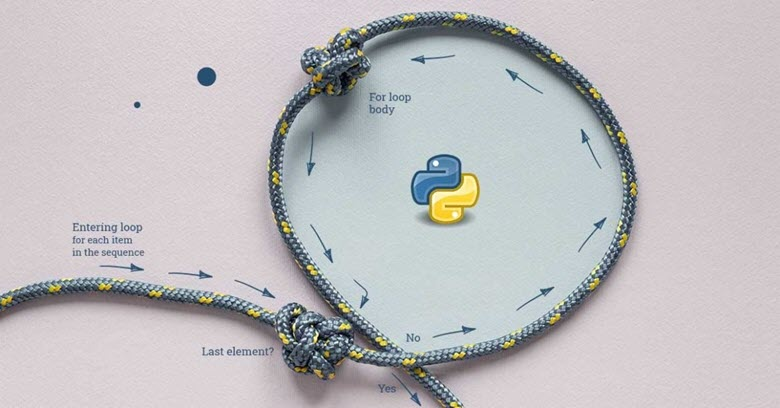## break

The break statement is responsible for terminating the loop that uses it. If the break statement is used in a nested loop, the current loop will terminate and the stream will continue to execute the code that follows the loop.

Flowchart of the break statement.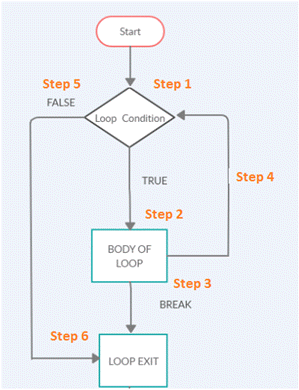Steps involved in the flowchart.

• Step 1) Loop execution starts.
• Step 2) If the loop condition is true, it will execute step 2, where the body of the loop will be executed.
• Step 3) If the body of the loop has a break statement, the loop will exit and go to step 6.
• Step 4) After the loop condition is executed and completed, it will go to the next iteration in step 4.
• Step 5) If the loop condition is false, it will exit the loop and go to step 6.
• Step 6) The loop ends.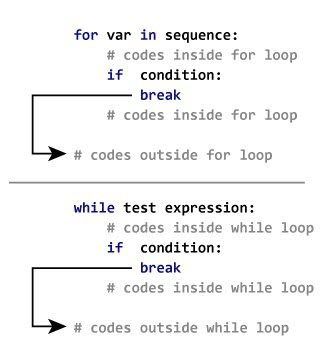### while loop

 `````` 1 2 3 4 5 6 7 8 9 10 11 12 `````` ``````my_list = ['Siya', 'Tiya', 'Guru', 'Daksh', 'Riya', 'Guru'] i = 0 while True: print(my_list[i]) if my_list[i] == 'Guru': print('Found the name Guru') break print('After break statement') i += 1 print('After while-loop exit') ``````

Output.

 ``````1 2 3 4 5 `````` ``````Siya Tiya Guru Found name Guru After while-loop exit ``````

When a break is encountered, the while loop is jumped out directly.

### for loop

 `````` 1 2 3 4 5 6 7 8 9 10 `````` ``````my_list = ['Siya', 'Tiya', 'Guru', 'Daksh', 'Riya', 'Guru'] for i in range(len(my_list)): print(my_list[i]) if my_list[i] == 'Guru': print('Found the name Guru') break print('After break statement') print('Loop is Terminated') ``````

Output content.

 ``````1 2 3 4 5 `````` ``````Siya Tiya Guru Found the name Guru Loop is Terminated ``````

When a break is encountered, the for loop is jumped out directly.

### Multi-level for loops

 ``````1 2 3 4 5 `````` ``````for i in range(4): for j in range(4): if j==2: break print("The number is ",i,j); ``````

Output.

 ``````1 2 3 4 5 6 7 8 `````` ``````The number is 0 0 The number is 0 1 The number is 1 0 The number is 1 1 The number is 2 0 The number is 2 1 The number is 3 0 The number is 3 1 ``````

Break will only jump out one layer.

## continue

When the continue statement is executed in the loop structure, it does not exit the loop structure, but immediately ends the current loop and starts the next loop again, that is, it skips all statements in the loop body after the continue statement and continues the next loop.

Flowchart of the continue statement.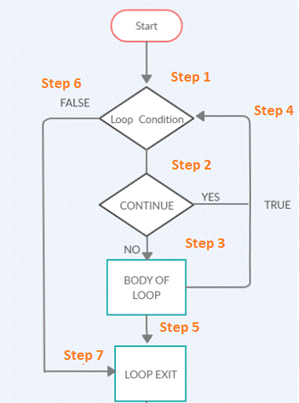Steps involved in the flowchart.

• Step 1) Loop execution begins.
• Step 2) Execution of the code within the loop will complete. If there is a continue statement in the loop, the control will return to step 4, the start of the next iteration of the loop.
• Step 3) Execution of the code within the loop will complete.
• Step 4) If there is a continue statement, or if the execution of the loop within the body completes, it will call the next iteration.
• Step 5) When the loop execution is complete, the loop will exit and go to step 7.
• Step 6) If the loop condition in step 1 fails, it will exit the loop and go to step 7.
• Step 7) The loop ends.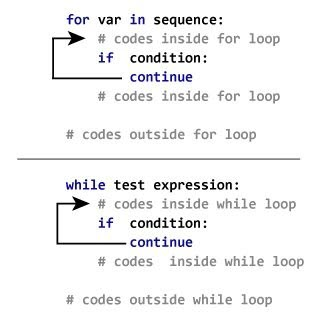### while loop

 ``````1 2 3 4 5 6 7 `````` ``````i = 0 while i <= 10: if i == 7: i += 1 continue print("The Number is :" , i) i += 1 ``````

Output.

 `````` 1 2 3 4 5 6 7 8 9 10 `````` ``````The Number is : 0 The Number is : 1 The Number is : 2 The Number is : 3 The Number is : 4 The Number is : 5 The Number is : 6 The Number is : 8 The Number is : 9 The Number is : 10 ``````

### for loop

 ``````1 2 3 4 `````` ``````for i in range(10): if i == 7: continue print("The Number is :" , i) ``````

Output.

 ``````1 2 3 4 5 6 7 8 9 `````` ``````The Number is : 0 The Number is : 1 The Number is : 2 The Number is : 3 The Number is : 4 The Number is : 5 The Number is : 6 The Number is : 8 The Number is : 9 ``````

### Multi-level for loops

 ``````1 2 3 4 5 `````` ``````for i in range(4): for j in range(4): if j==2: continue print("The number is ",i,j) ``````

Output.

 `````` 1 2 3 4 5 6 7 8 9 10 11 12 `````` ``````The number is 0 0 The number is 0 1 The number is 0 3 The number is 1 0 The number is 1 1 The number is 1 3 The number is 2 0 The number is 2 1 The number is 2 3 The number is 3 0 The number is 3 1 The number is 3 3 ``````

## pass

The Python pass statement is used as a placeholder in loops, functions, classes, and if statements, and does nothing; it is a null operation.

Usage scenario: Suppose you have a function or an empty class. You plan to write code in the future. If the Python interpreter encounters an empty object, it will throw an error. pass statements can be used inside function bodies or class bodies. During execution, when the interpreter encounters a pass statement, it ignores it and continues execution without giving any errors.